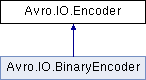Avro C#
Avro.IO.Encoder Interface Reference
Inheritance diagram for Avro.IO.Encoder:List of all members.

## Public Member Functions

void WriteNull ()
void WriteBoolean (bool value)
void WriteInt (int value)
void WriteLong (long value)
void WriteFloat (float value)
void WriteDouble (double value)
void WriteBytes (byte[] value)
void WriteString (string value)
void WriteEnum (int value)
void SetItemCount (long value)
void StartItem ()
void WriteArrayStart ()
void WriteArrayEnd ()
void WriteMapStart ()
void WriteMapEnd ()
void WriteUnionIndex (int value)
void WriteFixed (byte[] data)
void WriteFixed (byte[] data, int start, int len)

The documentation for this interface was generated from the following file:
• src/apache/main/IO/Encoder.cs